Multinomial Models

This type of model applies to cases where an observation can fall into one ofcategories. Binary data occur in the special case where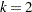. If there are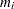observations in a subpopulation, then the probability distribution of the number falling into thecategories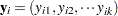can be modeled by the multinomial distribution, defined in the section Response Probability Distributions, with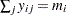. The multinomial model is an ordinal model if the categories have a natural order.

Residuals are not available in the OBSTATS table or the output data set for multinomial models.

By default, and consistently with binomial models, the GENMOD procedure orders the response categories for ordinal multinomial models from lowest to highest and models the probabilities of the lower response levels. You can change the way PROC GENMOD orders the response levels with the RORDER= option in the PROC GENMOD statement. The order that PROC GENMOD uses is shown in the "Response Profiles" output table described in the section Response Profile.

The GENMOD procedure supports only the ordinal multinomial model. If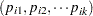are the category probabilities, the cumulative category probabilities are modeled with the same link functions used for binomial data. Let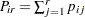,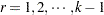, be the cumulative category probabilities (note that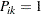). The ordinal model is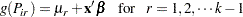where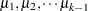are intercept terms that depend only on the categories and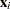is a vector of covariates that does not include an intercept term. The logit, probit, and complementary log-log link functionsare available. These are obtained by specifying the MODEL statement options DIST=MULTINOMIAL and LINK=CUMLOGIT (cumulative logit), LINK=CUMPROBIT (cumulative probit), or LINK=CUMCLL (cumulative complementary log-log). Alternatively,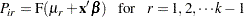where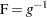is a cumulative distribution function for the logistic, normal, or extreme-value distribution.

PROC GENMOD estimates the intercept parametersand regression parametersby maximum likelihood.

The subpopulationsare defined by constant values of the AGGREGATE= variable. This has no effect on the parameter estimates, but it does affect the deviance and Pearson chi-square statistics; it also affects parameter estimate standard errors if you specify the SCALE=DEVIANCE or SCALE=PEARSON option.